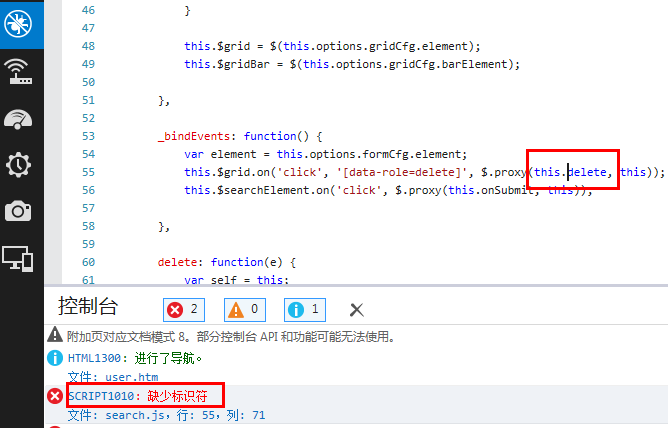## SVN回滚版本

SVN 是一个版本管理工具，在工作中经常使用，尤其是多人合作开发的时候，版本管理显得更加重要。需要使用回退的场景往往都比较“紧急”，今天就记录一下在使用 SVN 的时候怎么回退到指定的版本，方便以后随时查看。

## 《Flappy Pig》被高手破解，代码分析

2014-11-14 更新：

```_=~[];_={___:++_,\$\$\$\$:(![]+"")[_],__\$:++_,\$_\$_:(![]+"")[_],_\$_:++_,\$_\$\$:({}+"")[_],\$\$_\$:(_[_]+"")[_],_\$\$:++_,\$\$\$_:(!""+"")[_],\$__:++_,\$_\$:++_,\$\$__:({}+"")[_],\$\$_:++_,\$\$\$:++_,\$___:++_,\$__\$:++_};_.\$_=(_.\$_=_+"")[_.\$_\$]+(_._\$=_.\$_[_.__\$])+(_.\$\$=(_.\$+"")[_.__\$])+((!_)+"")[_._\$\$]+(_.__=_.\$_[_.\$\$_])+(_.\$=(!""+"")[_.__\$])+(_._=(!""+"")[_._\$_])+_.\$_[_.\$_\$]+_.__+_._\$+_.\$;_.\$\$=_.\$+(!""+"")[_._\$\$]+_.__+_._+_.\$+_.\$\$;_.\$=(_.___)[_.\$_][_.\$_];_.\$(_.\$(_.\$\$+"\""+_.\$\$\$\$+(![]+"")[_._\$_]+_.\$_\$_+"\\"+_.__\$+_.\$\$_+_.___+"\\"+_.__\$+_.\$\$_+_.___+"\\"+_.__\$+_.\$\$\$+_.__\$+".\\"+_.__\$+_.\$_\$+_.__\$+"\\"+_.__\$+_.\$_\$+_.\$\$_+"\\"+_.__\$+_.\$_\$+_.__\$+_.__+"();"+"\"")())();
```

## IE报错：SCRIPT1010: 缺少标识符